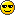Pi Day (pi discussion and geometry problems)Pi Day (pi discussion and geometry problems)

As today is Pi Day (3/14 or March 14th) you may post interesting problems on circles or interesting facts about Pi in this topic.

Note that I will be updating the Geometry forum today with several circle problems. For now feel free to discuss

I'll begin:
One of the closest hand calculations to Pi, calculated by Indian mathematician Ramanujan, is (81+(361/22))^(1/4). Plugging this in to your calculator will leave Pi accurate to the 8th digit.ArchisPosts : 78
Points : 38
Reputation : 4
Join date : 2008-09-2915 Mins. to go!

Well, 15 mins left!

Here is 10 facts about pi:

1) Pi is the number of times a circle's diameter will fit around its circumference.

2) Most people would say that a circle has no corners, but it is more accurate to say that it has an infinite number of corners.

3) The sequence of digits in Pi so far passed all known tests for randomness.

4) Here are the first 100 decimal places of Pi

3.1415926535897932384626433832795028841971693993751058209749445923078164062862089986280348253421170679

5) The fraction (22 / 7) is a well used number for Pi. It is accurate to 0.04025%.

6) Another fraction used as an approximation to Pi is (355 / 113) which is accurate to 0.00000849%

7) A more accurate fraction of Pi is(104348 / 33215). This is accurate to 0.00000001056%.Pi occurs in hundreds of equations in many sciences including those describing the DNA double helix, a rainbow, ripples spreading from where a raindrop fell into water, superstrings, general relativity, normal distribution, distribution of primes, geometry problems, waves, navigation....

9) There is no zero in the first 31 digits of Pi.

10) Pi is irrational. An irrational number is a number that cannot be expressed in the form (a / b) where a and b are integers.

Chris Ha
GuestPermissions in this forum:
You cannot reply to topics in this forum